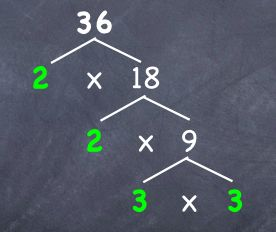Learn the 4 Things You Must Know Before Taking the GMAT!   Download it Now!

# GMAT Prime Factorization – A Primer

Prime factorization is a strategy that has great application on both GMAT problem solving and GMAT data sufficiency questions, and yet it’s often under-utilized by students for whatever reason. Hopefully the better you understand it, the more it will come to mind for you as a possible solution method — especially on more difficult questions!

### What is Prime Factorization?

Prime Factorization is simply a process of breaking a number down into its most basic, prime number factors.

Remember that a prime number is a number that is only divisible by 1 and itself. The first 10 prime numbers are: 2, 3, 5, 7, 11, 13, 17, 19, 23, and 29.

To find the prime factors of a number (i.e. to find a number’s prime factorization), divide the number by the primes in order, starting with 2, and use each repeatedly until it is no longer a factor.

For example,

What is the prime factorization of 36?

Well, 36 is the same as 2 x 18. Then, 18 can be further factored as 2 x 9. Now 2 no longer factors evenly into 9, but 3 does. So, 3 x 3 = 9. And there you have it! The prime factorization of 36 is 2 x 2 x 3 x 3. It can be represented this way (graphic below), which is a good way to draw out the prime factorization of a number on your scratch paper on test day:### How is Prime Factorization useful on the GMAT?

Now that you know how to find the prime factorization of a number, when would you actually use it on the GMAT? Truthfully, it should be part of your thought process on any GMAT problem solving or data sufficiency questions that talk about products or multiples or factors or prime numbers.

Consider this example from the GMAT Review Official Guide, 14th Edition (#219, p.183):

In a certain game, a large container is filled with red, yellow, green, and blue beads worth, respectively, 7, 5, 3, and 2 points each. A number of beads are then removed from the container. If the product of the point values of the removed beads is 147,000, how many red beads were removed?

(A) 5
(B) 4
(C) 3
(D) 2
(E) 0

If you had trouble with this question, fear not! It’s an admittedly difficult GMAT sample question. That said, did you think about using prime factorization to solve it? One tip-off in the question that should have made you think about using prime factorization are the words “If the product of … is 147,000…?”

So, what prime factors, when multiplied together, would equal 147,000? If you remember my analogy of “driving through the fog with headlights,” this is a great “next step” to do on your scratch paper — find the prime factorization of 147,000!

Sure enough, you find that 147,000 = 2 x 2 x 2 x 3 x 5 x 5 x 5 x 7 x 7

Now, the question asks us how many red beads were removed. Red beads are associated with a point value of 7. As we see from our prime factorization, there are only two (2) factors of 7 in 147,000. Thus, the only way we could get that score of 147,000 is if two (2) red beads are removed! That’s it! The answer is D, 2.

To see how you might approach this question on your own scratch paper on test day, check out this solution video. Note: You’ll want to fast-forward to the 4:25 mark to see this particular problem explained.